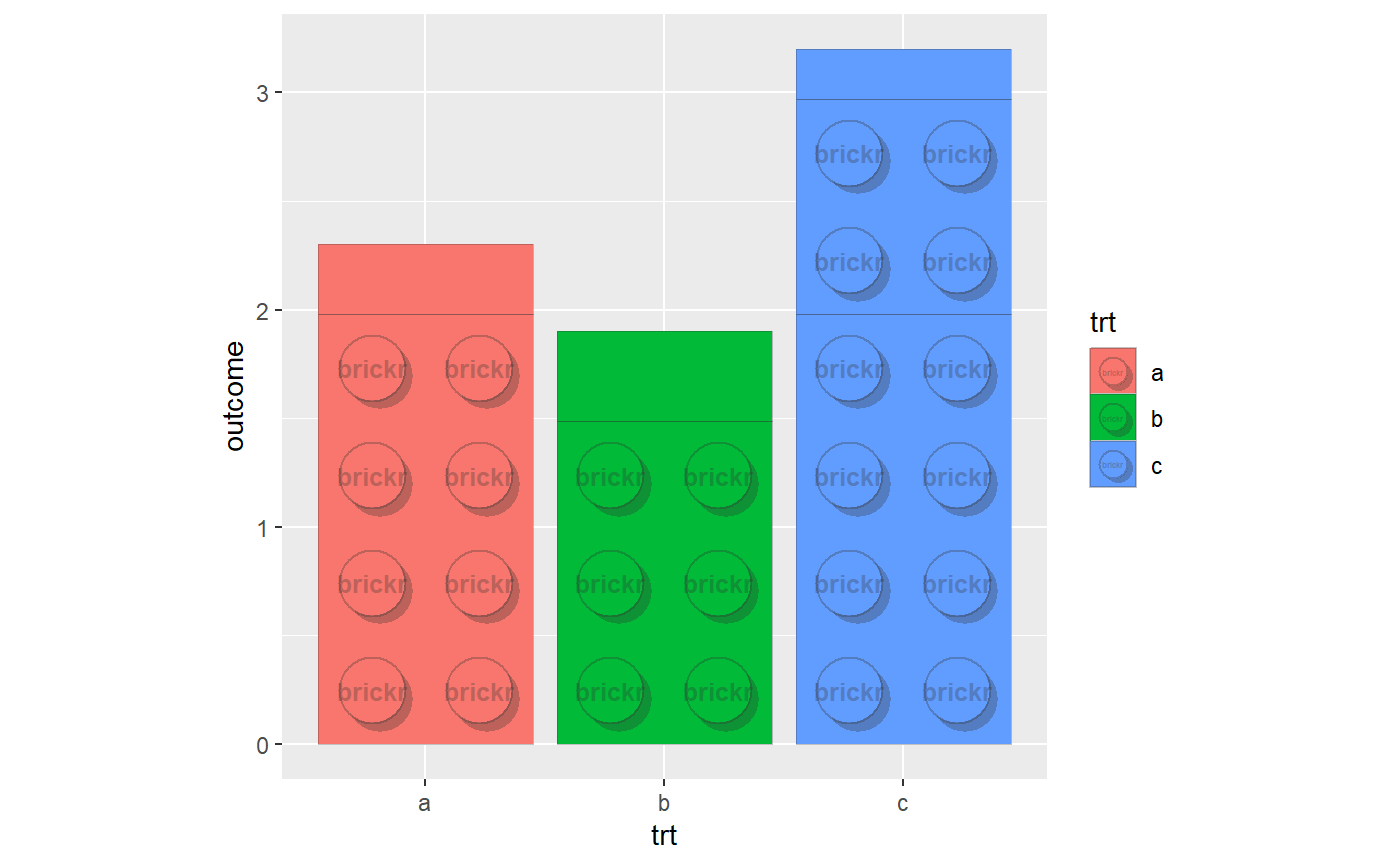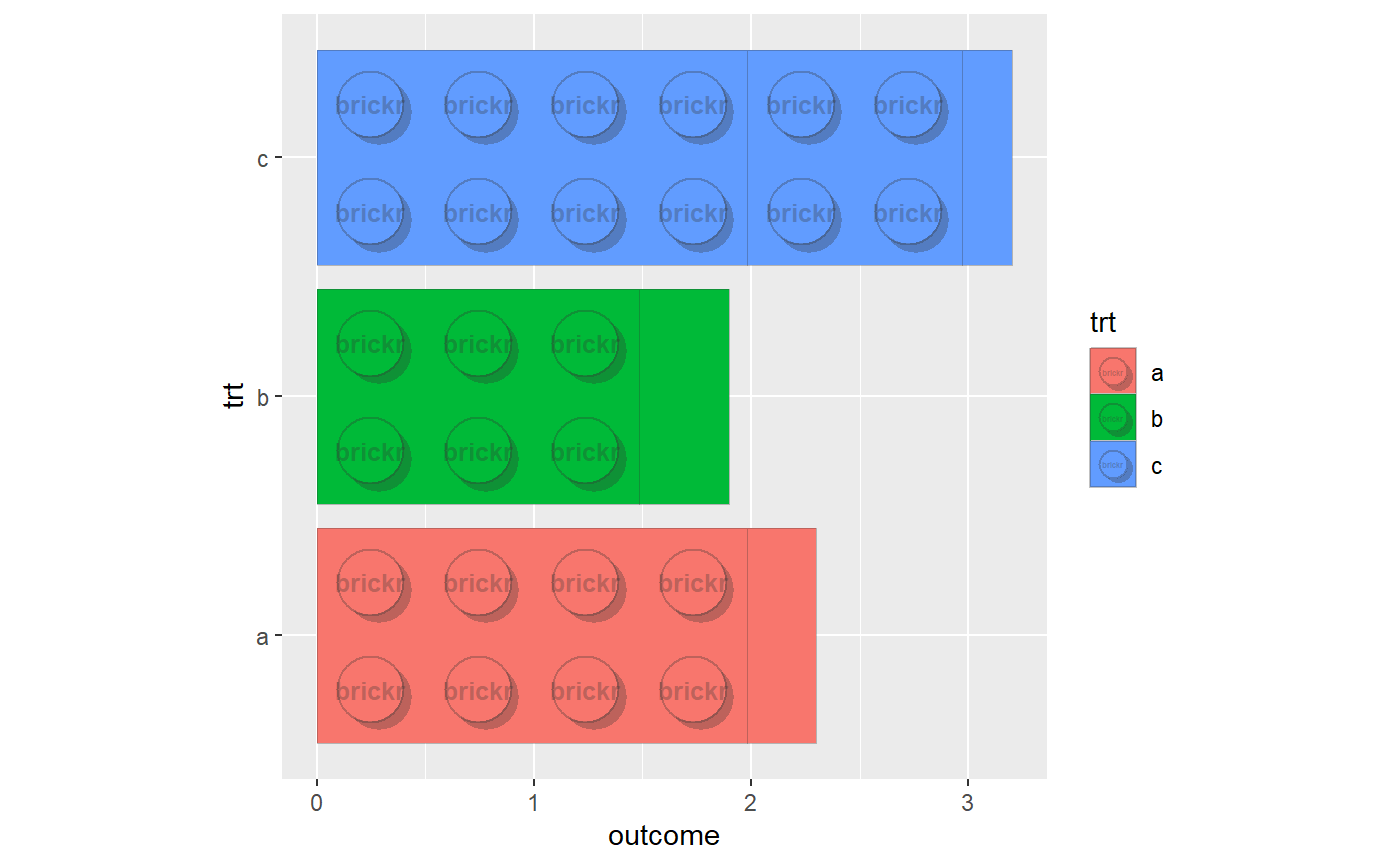A fixed scale coordinate system that ensures correct brick proportions are maintained regardless of device size. Use coord_brick_flip() for horizontal bars.

coord_brick(xlim = NULL, ylim = NULL, expand = TRUE, clip = "on")

coord_brick_flip(xlim = NULL, ylim = NULL, expand = TRUE,
clip = "on")

## Arguments

xlim Limits for the x and y axes. Limits for the x and y axes. If TRUE, the default, adds a small expansion factor to the limits to ensure that data and axes don't overlap. If FALSE, limits are taken exactly from the data or xlim/ylim. Should drawing be clipped to the extent of the plot panel? A setting of "on" (the default) means yes, and a setting of "off" means no. In most cases, the default of "on" should not be changed, as setting clip = "off" can cause unexpected results. It allows drawing of data points anywhere on the plot, including in the plot margins. If limits are set via xlim and ylim and some data points fall outside those limits, then those data points may show up in places such as the axes, the legend, the plot title, or the plot margins.

Other Graphs: geom_brick_col, geom_brick_rect, scale_fill_brick, theme_brick

## Examples

#geom_brick_col should be used in conjunction with other brickr charting
#functions, especially coord_brick.
df <- data.frame(trt = c("a", "b", "c"), outcome = c(2.3, 1.9, 3.2))
ggplot(df, aes(trt, outcome)) +
geom_brick_col(aes(fill = trt)) +
coord_brick()#horizontal bars
ggplot(df, aes(trt, outcome)) +
geom_brick_col(aes(fill = trt)) +
coord_brick_flip()